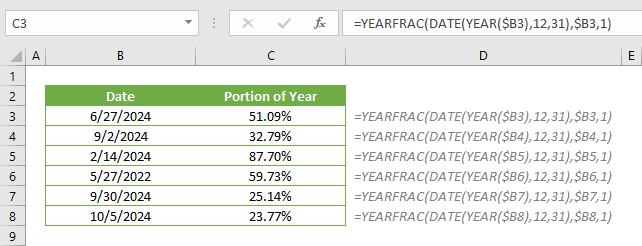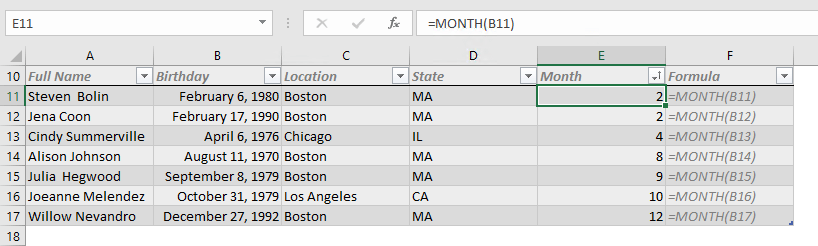## How to calculate the percent of completed year

A percentage value is a great way to express a proportion’s size in whole. You may want to calculate and see what percent of the year is completed. In this article, we are going to show you how to calculate the percent of completed year in Excel. Download Workbook...## How to sort dates by month in Excel

Sorting is a crucial part of organizing data. Being everyone’s go-to spreadsheet tool, Excel thankfully offers various tools for sorting data like the sort feature and functions like SORT or SORTBY. However, neither of these approaches can really help you with...## How to create an Excel summary table using UNIQUE and SUMIFS

SUMIFS like other …IF or …IFS function are great tools to aggregate data based on a set of conditions. The downside of this approach is that the criteria must be supplied manually, especially when you need to create a summary table. In this guide, we’re going to show...## How to do a cell count if not equal to a variable or even an error code

You can use the COUNTIFS function to count the values in ranges that meet certain criteria. The criteria can contain a not equal logic as well. However, the COUNTIFS function requires individual arguments for each range-criteria pair. This requirement can make it...## How to count if not equal to x or y in Excel

The COUNTIFS function can count the values in multiple ranges with multiple criteria. The function counts each given range-criteria pair and combines the result with AND logical operator. Unfortunately, there is no quick and easy way to use an OR logic with the...## How to make complex formulas easier to read with line breaks in Excel

Excel allows you to enter a formula with up to 8,192 characters. However, managing a formula will certainly get harder as you get closer to that limit. You might especially have this issue when working with nested formulas – functions in functions. In this...## How to analyze complex formulas with the Excel Evaluate formula feature

Are extremely long, formulas nested arguments intimidating you? The Evaluate Formula feature can help you untangle these complex formulas! This tool essentially solves complex formulas step by step. In this guide, we’re going to show you how to step through...